PSA6P2D - Practice Problems---7.1 Lesson 4: Scaled Relationships (7.G.A.1)

Select all the statements that must be true for any scaled copy Q of Polygon P.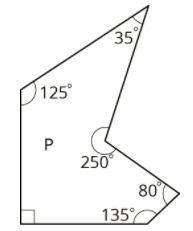Select all that apply:
Part A)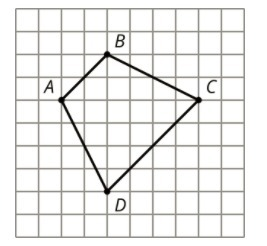Quadrilateral PQRS is a scaled copy of Quadrilateral ABCD. Point P corresponds to AQ to BR to C, and S to D.

If the distance from P to R is 3 units, what is the distance from Q to S?

Type your answer below as a number (example: 5, 3.1, 4 1/2, or 3/2):
Part B)

Part A)

Figure 2 is a scaled copy of Figure 1.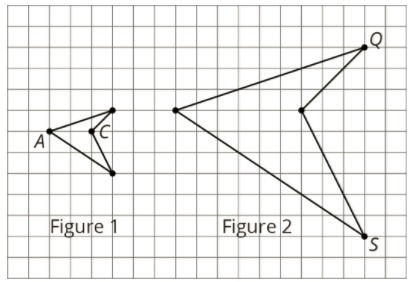Identify the points in Figure 2 that correspond to the points A and C in Figure 1. Label them P and R.

Label your points on paper, take a picture, and upload it using the image upload icon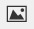If you do not have the ability to upload an image of your work type "Figure 2 is on paper."

Part B)

Figure 2 is a scaled copy of Figure 1.What is the distance between P and R?

Type your answer below as a number (example: 5, 3.1, 4 1/2, or 3/2):
Part C)

Figure 2 is a scaled copy of Figure 1.Identify the points in Figure 1 that correspond to the points Q and S in Figure 2. Label them B and D.

Label your points on paper, take a picture, and upload it using the image upload iconIf you do not have the ability to upload an image of your work type "Figure 2 is on paper."

Part D)

Figure 2 is a scaled copy of Figure 1.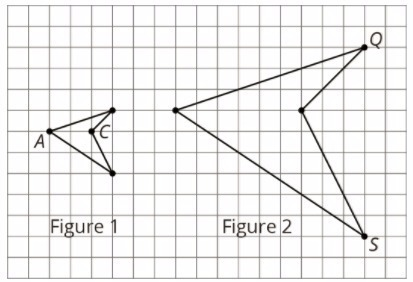What is the distance between B and D?

Type your answer below as a number (example: 5, 3.1, 4 1/2, or 3/2):
Part E)

Figure 2 is a scaled copy of Figure 1.What is the scale factor that takes Figure 1 to Figure 2?

Type your answer below as a number (example: 5, 3.1, 4 1/2, or 3/2):
Part F)

Figure 2 is a scaled copy of Figure 1.G and H are two points on Figure 1, but they are not shown. The distance between and H is 1. What is the distance between the corresponding points on Figure 2?